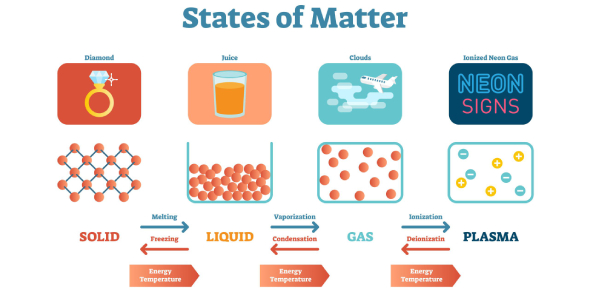# Trivia: Questions On States Of Matter! Quiz

13 Questions | Total Attempts: 1026Settings.

Related Topics
• 1.
The amount of energy needed to change a substance from a solid phase to a liquid phase is __________.
• A.

Heat of transformation

• B.

Heat of fusion

• C.

Melting point

• D.

Heat of vaporization

• 2.
__________ is the energy an object has due to its motion.
• A.

Kinetic energy

• B.

Diffusion

• C.

Heat of fusion

• D.

Heat of vaporization

• 3.
When the temperature of a substance is lowered, its particles __________ .
• A.

Escape the attractive forces of the other particles

• B.

Vibrate more slowly

• C.

Stop vibrating completely

• D.

Vibrate more quickly

• 4.
Which of the following is a state of matter?
• A.

Particle

• B.

Diffuse

• C.

Plasma

• D.

Thermal

• 5.
What is the most common state of matter in the universe?
• A.

Solid

• B.

Liquid

• C.

Gas

• D.

Plasma

• 6.
The states of matter are the following: solids, liquids, gases, plastics.
• A.

True

• B.

False

• 7.
There is very little space between the molecules of a liquid. There is a lot of space between the molecules of a gas.
• A.

True

• B.

False

• 8.
Liquids expand in all directions to take the shape of the container it is in.
• A.

False

• B.

True

• 9.
Compression forces atoms closer together. Compression forces something into a smaller space.
• A.

True

• B.

False

• 10.
When solids reach their melting points, solids become liquids
• A.

True

• B.

False

• 11.
Liquids do not have a set shape.
• A.

True

• B.

False

• 12.
All liquids freeze at the same temperature.
• A.

True

• B.

False

• 13.
Gases have no weight.
• A.

True

• B.

False